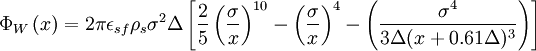# 10-4-3 Lennard-Jones potential

Jump to: navigation, searchThis article is a 'stub' page, it has no, or next to no, content. It is here at the moment to help form part of the structure of SklogWiki. If you add sufficient material to this article then please remove the {{Stub-general}} template from this page.

The 10-4-3 model is a variant of the Lennard-Jones model designed for use in fluid-wall systems and is given by$\Phi_{W} \left( x \right) = 2\pi \epsilon_{sf} \rho_s \sigma^2 \Delta \left[ \frac{2}{5} \left( \frac{ \sigma} { x }\right)^{10} - \left( \frac{ \sigma} { x }\right)^{4} - \left( \frac{\sigma^4 }{ 3\Delta( x + 0.61 \Delta)^3 }\right) \right]$